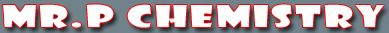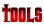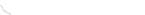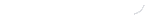activity seriesbond energybond lengthscalculatorcommon namesconversion factors: energyconversion factors: lengthconversion factors: massconversion factors: pressureconversion factors: temp.conversion factors: volumecovalent prefixesdensity solverdiatomic elementse-config. chartelement listgas law formulasKa's of polyprotic acidsKa's of weak acidsKb's of weak basesmetric conversion chartmole conversion chartmolecular geometriesorganic prefixesperiodic tableperiodic table (flash)pH/pOH converterpolyatomic ionspressure converterSI unitssolubility chartsolubility of salts rulessolubility product constantsstoichiometry charttemp. conversiontemp. formulasthermodynamic datavapor pressure of water
How To Round Numbers
 Rule 1. If the digit following the last significant figure is less than 5, the last significant figure remains unchanged. The digits after the last significant figure are dropped. Example: Round 23.437 to three significant figures. Answer: 23.4 Explanation: 4 is the last significant figure. The next number is 3. 3 is less than 5. Thus, 4 remains unchanged and 37 is dropped. Rule 2. If the digit following the last significant figure is greater than 5, then 1 is added to the last significant figure. The digits after the last significant figure are dropped. Example: Round 5.383 to two significant figures. Answer: 5.4 Explanation: 3 is the last significant figure. The next number is 8. 8 is greater than 5. Thus, 1 is added to 3 making it 4. The 83 is dropped. Rule 3. If the digit following the last significant figure is 5, then 1 is added to the last significant figure if the last significant figure is odd. If the last significant figure is even, it remains unchanged and the digits after the last significant figure are dropped. Example 1: Round 3.35 to two significant figures. Answer: 3.4 Explanation: 3 is the last significant figure. The next number is 5. 3 is an odd number. Odd numbers round up when the following number is a 5. Example 2: Round 7.25 to two significant figures. Answer: 7.2 Explanation: 2 is the last significant figure. The next number is 5. 2 is an even number. Even numbers remain unchanged when they are followed by a five. The 5 is dropped.

 You may also find these related tutorials helpfulCompounds in Crust of EarthDensityElements in Crust of EarthHow To Round NumbersMetric ConversionScientific NotationSI UnitsSignificant Figure CalculationsSignificant Figures